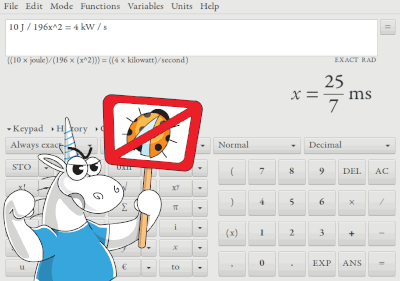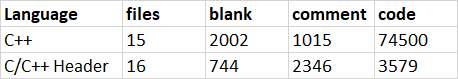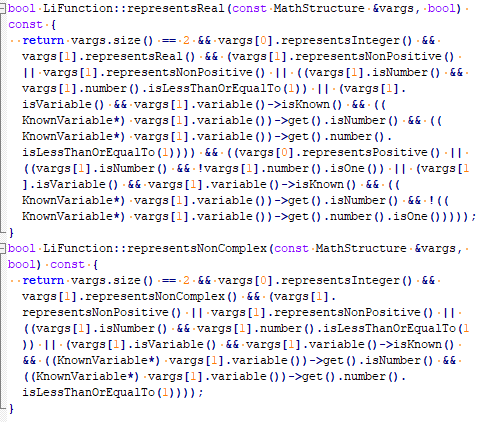﻿ Following in the Footsteps of Calculators: Qalculate!# Following in the Footsteps of Calculators: Qalculate!

Previously we did code reviews of large mathematical packages, for example, Scilab and Octave, whereby calculators remained aloof as small utilities, in which it is difficult to make errors due to their small codebase. We were wrong that we haven't paid attention to them. The case with posting the source code of the Windows calculator showed that actually everyone was interested in discussing types of errors hiding in it. Moreover, the number of errors there was more than enough to write an article about that. My colleagues and I, we decided to explore the code of a number of popular calculators, and it turned out that the code of the Windows calculator was not that bad (spoiler).## Introduction

Qalculate! is a multi-purpose cross-platform desktop calculator. It is simple to use but provides power and versatility normally reserved for complicated math packages, as well as useful tools for everyday needs (such as currency conversion and percent calculation). The project consists of two components: libqalculate (library and CLI) and qalculate-gtk (GTK + UI). The study involved only the libqalculate code.

To easily compare the project with Windows Calculator, which we have recently checked, I'm citing the output of the Cloc utility for libqalculate:Considering it subjectively, there are more errors in it and they are more critical than in the Windows calculator code. Nevertheless, I would recommend making conclusions on your own, having read this code overview.

Bug reviews for the other projects:

The analysis tool is the PVS-Studio static code analyzer. It is a set of solutions for code quality control, search for bugs and potential vulnerabilities. Supported languages include: C, C++, C# and Java. You can run the analyzer on Windows, Linux and macOS.

## Copy-paste and Typos Again!

V523 The 'then' statement is equivalent to the 'else' statement. Number.cc 4018

``````bool Number::square()
{
....
if(mpfr_cmpabs(i_value->internalLowerFloat(),
i_value->internalUpperFloat()) > 0) {
mpfr_sqr(f_tmp, i_value->internalLowerFloat(), MPFR_RNDU);
mpfr_sub(f_rl, f_rl, f_tmp, MPFR_RNDD);
} else {
mpfr_sqr(f_tmp, i_value->internalLowerFloat(), MPFR_RNDU);
mpfr_sub(f_rl, f_rl, f_tmp, MPFR_RNDD);
}
....
}``````

The code is absolutely the same in the if and else blocks. Adjacent code fragments are very similar to this one, but different functions are used in them: internalLowerFloat() and internalUpperFloat(). It is safe to assume that a developer copied the code and forgot to correct the name of the function here.

V501 There are identical sub-expressions '!mtr2.number().isReal()' to the left and to the right of the '||' operator. BuiltinFunctions.cc 6274

``````int IntegrateFunction::calculate(....)
{
....
if(!mtr2.isNumber() || !mtr2.number().isReal() ||
!mtr.isNumber() || !mtr2.number().isReal()) b_unknown_precision = true;
....
}``````

In this case, duplicated expressions appeared due to the fact that in one place mtr2 was written instead of mtr. Thus, a call of the mtr.number().isReal() function is absent in the condition.

V501 There are identical sub-expressions 'vargs.representsNonPositive()' to the left and to the right of the '||' operator. BuiltinFunctions.cc 5785We would have never found defects in this code manually! But here there are. Moreover, in the original file these fragments are written in a single line. The analyzer has detected a duplicated expression vargs.representsNonPositive(), which may indicate a typo or, consequently, a potential error.

Here's the entire list of suspicious places, which one can barely puzzle out.

• V501 There are identical sub-expressions 'vargs.representsNonPositive()' to the left and to the right of the '||' operator. BuiltinFunctions.cc 5788
• V501 There are identical sub-expressions 'append' to the left and to the right of the '&&' operator. MathStructure.cc 1780
• V501 There are identical sub-expressions 'append' to the left and to the right of the '&&' operator. MathStructure.cc 2043
• V501 There are identical sub-expressions '(* v_subs[v_order]).representsNegative(true)' to the left and to the right of the '&&' operator. MathStructure.cc 5569

## Loop With Incorrect Condition

V534 It is likely that a wrong variable is being compared inside the 'for' operator. Consider reviewing 'i'. MathStructure.cc 28741

``````bool MathStructure::isolate_x_sub(....)
{
....
for(size_t i = 0; i < mvar->size(); i++) {
if((*mvar)[i].contains(x_var)) {
mvar2 = &(*mvar)[i];
if(mvar->isMultiplication()) {
for(size_t i2 = 0; i < mvar2->size(); i2++) {
if((*mvar2)[i2].contains(x_var)) {mvar2 = &(*mvar2)[i2]; break;}
}
}
break;
}
}
....
}``````

In the inner loop, the i2 variable represents a counter, but due to a typo an error was made - the i variable from the outer loop is used in the loop exit condition.

## Redundancy or an Error?

V590 Consider inspecting this expression. The expression is excessive or contains a misprint. Number.cc 6564

``````bool Number::add(const Number &o, MathOperation op)
{
....
if(i1 >= COMPARISON_RESULT_UNKNOWN &&
(i2 == COMPARISON_RESULT_UNKNOWN || i2 != COMPARISON_RESULT_LESS))
return false;
....
}``````

3 years ago after I got an eyeful of such code, I wrote a cheat sheet for me and other developers: "Logical Expressions in C/C++. Mistakes Made by Professionals". When I come across such code, I make sure that the note hasn't become less relevant. You can look into the article, find a pattern of the error corresponding to the code, and find out all the nuances.

In the case of this example, we'll go to the section "Expression == || !=" and find out that the expression i2 == COMPARISON_RESULT_UNKNOWN affects nothing.

## Dereferencing Unchecked Pointers

V595 The 'o_data' pointer was utilized before it was verified against nullptr. Check lines: 1108, 1112. DataSet.cc 1108

``````string DataObjectArgument::subprintlong() const {
string str = _("an object from");
str += " \"";
str += o_data->title();               // <=
str += "\"";
DataPropertyIter it;
DataProperty *o = NULL;
if(o_data) {                          // <=
o = o_data->getFirstProperty(&it);
}
....
}``````

In one function the o_data pointer is dereferenced both without and with a check. This can be redundant code, or a potential error. I'm leaning toward the latter.

There are two similar places:

• V595 The 'o_assumption' pointer was utilized before it was verified against nullptr. Check lines: 229, 230. Variable.cc 229
• V595 The 'i_value' pointer was utilized before it was verified against nullptr. Check lines: 3412, 3427. Number.cc 3412

## free() or delete []?

V611 The memory was allocated using 'new' operator but was released using the 'free' function. Consider inspecting operation logics behind the 'remcopy' variable. Number.cc 8123

``````string Number::print(....) const
{
....
while(!exact && precision2 > 0) {
if(try_infinite_series) {
remcopy = new mpz_t;                          // <=
mpz_init_set(*remcopy, remainder);
}
mpz_mul_si(remainder, remainder, base);
mpz_tdiv_qr(remainder, remainder2, remainder, d);
exact = (mpz_sgn(remainder2) == 0);
if(!started) {
started = (mpz_sgn(remainder) != 0);
}
if(started) {
mpz_mul_si(num, num, base);
}
if(try_infinite_series) {
if(started && first_rem_check == 0) {
remainders.push_back(remcopy);
} else {
if(started) first_rem_check--;
mpz_clear(*remcopy);
free(remcopy);                                 // <=
}
}
....
}
....
}``````

The memory for the remcopy array is allocated and released in different ways, which is a serious error.

## Lost Changes

V672 There is probably no need in creating the new 'm' variable here. One of the function's arguments possesses the same name and this argument is a reference. Check lines: 25600, 25626. MathStructure.cc 25626

``````bool expand_partial_fractions(MathStructure &m, ....)
{
....
if(b_poly && !mquo.isZero()) {
MathStructure m = mquo;
if(!mrem.isZero()) {
m += mrem;
m.last() *= mtest[i];
m.childrenUpdated();
}
expand_partial_fractions(m, eo, false);
return true;
}
....
}``````

The m variable in the function is passed by reference, which means its modification. However, the analyzer has detected that the code contains the variable with the same name, which overlaps the scope of function's parameter, allowing for loss of changes.

## Strange Pointers

V774 The 'cu' pointer was used after the memory was released. Calculator.cc 3595

``````MathStructure Calculator::convertToBestUnit(....)
{
....
CompositeUnit *cu = new CompositeUnit("", "....");
Unit *u = getBestUnit(cu, false, eo.local_currency_conversion);
if(u == cu) {
delete cu;                                   // <=
return mstruct_new;
}
delete cu;                                     // <=
if(eo.approximation == APPROXIMATION_EXACT &&
cu->hasApproximateRelationTo(u, true)) {    // <=
if(!u->isRegistered()) delete u;
return mstruct_new;
}
....
}``````

The analyzer warns that the code calls a method of the cu object right after deallocating memory. But when trying to grapple with it, the code turns out to be even more strange. Firstly, calling delete cu happens always - both in the condition and after that. Secondly, the code after the condition implies that the pointers u and cu are not equal, which means that after deleting the cu object it is quite logical to use the u object. Most likely, a typo was made in the code and the author of the code wanted to use only the u variable.

## Usage of the find Function

V797 The 'find' function is used as if it returned a bool type. The return value of the function should probably be compared with std::string::npos. Unit.cc 404

``````MathStructure &AliasUnit::convertFromFirstBaseUnit(....) const {
if(i_exp != 1) mexp /= i_exp;
ParseOptions po;
if(isApproximate() && suncertainty.empty() && precision() == -1) {
if(sinverse.find(DOT) || svalue.find(DOT))
}
....
}``````

Even though the code can be successfully compiled, it looks suspicious, as the find function returns the number of the type std::string::size_type. The condition will be true if the point is found in any part of the string except if the point is at the beginning. It is a strange check. I'm not sure but, perhaps, this code should be rewritten as follows:

``````if(   sinverse.find(DOT) != std::string::npos
||   svalue.find(DOT) != std::string::npos)
{
}``````

## Potential Memory Leak

V701 realloc() possible leak: when realloc() fails in allocating memory, original pointer 'buffer' is lost. Consider assigning realloc() to a temporary pointer. util.cc 703

``````char *utf8_strdown(const char *str, int l) {
#ifdef HAVE_ICU
....
outlength = length + 4;
buffer = (char*) realloc(buffer, outlength * sizeof(char)); // <=
....
#else
return NULL;
#endif
}``````

When working with the realloc() function it is recommended to use an intermediate buffer, as in case if it is impossible to allocate memory, the pointer to the old memory area will be irretrievably lost.

## Conclusion

The Qalculate! project tops the list of the best free calculators, whereas it contains many serious errors. On the other hand, we haven't checked out its competitors yet. We'll try to go over all popular calculators.

As for comparing with the quality of the calculator from the Windows world, the utility from Microsoft looks more reliable and well-worked so far.##### Use PVS-Studio to search for bugs in C, C++, C# and Java

We offer you to check your project code with PVS-Studio. Just one bug found in the project will show you the benefits of the static code analysis methodology better than a dozen of the articles.

381
13 764